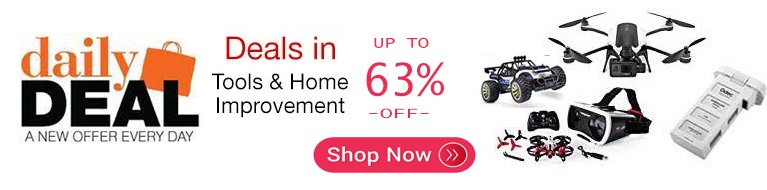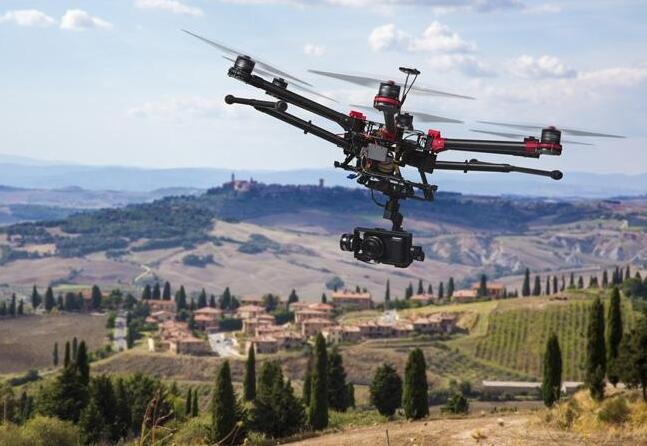# How to calculate quadcopter power consumption

You want to know how to calculate quadcopter power consumption when trying to figure out the propulsion system or building a quadcopter, here you come to the right place.

## How to calculate a quadcopter power consumption

### #2 Take an example

Drone requirements from a student:

I am currently looking at the design of a drone for use with emergency response services, in particular, the ambulance service.

I am currently trying to figure out the propulsion system. I know the AUW will be around 7kg, I need to achieve a speed of around 40ms and am planning on using a hexacopter. I was wondering if you had any advice on what size props/motors I should be using? or the calculations I should be looking at?

Analysis (only for reference):

If AUW is around 7000g, 2:1 thrust ratio so 14000g, divided by 4 motors = 3500g per motor. oh no, it’s a overkill weigh for 4 motors. so you can use 6 motors then 2300g thrust per motor (14000g / 6). Then you can pick up motors and props as below.

Motor: 4240 Brushless Motor 1300kv

Voltage: 3-4S

Max current: 50~60A/20S

Prop: 10×5

Thrust: 1860~2400gOther examples from Internet:

Example 1:

One of my motors draws 9 amps continuously at 65% power.

I have four motors so 9 times 4 is 36. So 36 total amps being drawn from the battery.

Equation: (36 x 1000)/60 = 600 mah’s consumed per minute.

I have a 9000 mah battery. So 9000/600 = 15 minutes of 65% continuous power usage.

Example 2:

Amps x Volts = Watts sport planes are about 100w per lb….that is around 10a or so on a 3s battery for example

Like the guys said above there are a lot of variables in a quad. I have found you cant go by amps or watts quite the same as a plane. You need to know your all up weight AUW and then you know how much thrust you need to make it fly

If your all up weight is 1000g you want a min of 2:1 thrust ratio so 2000g…..divided by 4 motors = 500g per motor….what size props can you run? what size esc’s do you have? Then you can pick motor size and kv and amps

One of my quads had 2200kv motors that handle 34amps, with a 8×4.5 props each motor would pull 33a WOT and 1300g of thrust…..way overkill for a 900g quad….need a lot of battery to handle the 100a and flight times were short

I then stuck 980kv motors that only handle 16a with the same props….only pulled 10a each with 550g of thrust….more than 2:1….much less power but flies nice and flight times almost doubled

Example 3:

I written a article about how to calculate quadcopter flight time,if know your quadcopter battery capacity,battery discharge and quadcopter average amp draw, you can calculate flight times by this formula (Battery Capacity * Battery Discharge /Average Amp Draw)*60 (Link:calculate quadcopter flight time).

If you know each motor draws 1 amps to produce 100g of thrust. if your quadcopter take 2000g to flight, each motor will have to produce 500g (500g = 2000g / 4 motors) of thrust in order to hover. Then your quadcopter Average Amp Draw is 20AMPS ( 20AMPS =4motors * 500g/100g per 1amps);

## Famous quadcopter power consumption comparsion chart

 Drone Power consumption Flight Time Take-off Weight Phantom 2 5.6W 25 mins 1.3kg MAX Typhoon Q500 10W 25 mins 1.7kg Typhoon H 16W 22 mins 1.8kg MG-1 6400 W 10 mins 22.5 kg